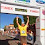## Tuesday, February 09, 2010

A confused bank teller transposed the dollars and cents when he cashed a check for Ms Smith, giving her dollars instead of cents and cents instead of dollars. After buying a newspaper for 50 cents, Ms Smith noticed that she had left exactly three times as much as the original check. What was the amount of the check?

1.2.we ARE extremely rusty in our
algebraic skills. :-)

we spent an inordinate (pun!)
amount of time juggling
x's, y', a' & b's with nothing
to show for it except a proper humbling.
{our guess is that it was overdue}

we were able, however,
to confirm Andy's answer through other means.

it appears that \$18.56 is correct. kudos!

and thanks for the twister

..
.ero

3.Let x be the number of dollars in the check, and y be the number of cents.

Then 100y + x − 50 = 3(100x + y).

Therefore 97y − 299x = 50.

Keep in mind that x and y have to be between 0 and 100

It can be shown that all integer solutions of 97y − 299x = 50 are of the form y = 1850 + 299k, x = 600 + 97k, where k is any integer. (I'm not going to show this... too much writing!)

In this case, because x and y must be between 0 and 99, we choose k = −6.

This gives y = 56, x = 18.

So the check was for \$18.56.

4.In case anyone cares to know, the way I found this one was sort of a brute force method. I got the equation that Mike mentioned, and solved for x:

x = (97y - 50)/299

Then I used a quick spreadsheet to find x for all possible values of y (00...99), and found that x=18, y=56 was the only one that came out to integer values, so that was my answer.

Not sure if the spreadsheet is considered cheating though :)

Leave your answer or, if you want to post a question of your own, send me an e-mail. Look in the about section to find my e-mail address. If it's new, I'll post it soon.

Please don't leave spam or 'Awesome blog, come visit mine' messages. I'll delete them soon after.

Enter your Email and join hundreds of others who get their Question of the Day sent right to their mailbox

The Lamplight Manor Puzz 3-D